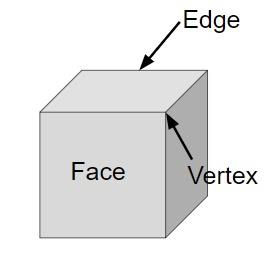# Program for Volume and Surface Area of Cube in C++

## What is cube?

Cube is a three-dimensional object with six faces of square shape which means it has sides of same length and breadth. Cube is the only regular hexahedron with following properties −

• six faces
• 12 edges
• 8 vertices

Given below is the figure of cube## Problem

Given with the side, the task is to find the total surface area and volume of a cube where surface area is the space occupied by the faces and volume is the space that a shape can contain.

To calculate surface area and volume of a cube there is a formula −

Surface Area = 6*Side*side

Volume = Side*side*side

## Example

Input-: side=3
Output-: volume of cube is: 27
Total surface area of cube is 54

## Algorithm

Start
Step 1 -> declare function to find volume of cube
double volume(double a)
return (a*a*a)
Step 2 -> declare function to find area of cube
double volume(double a)
return (6*a*a)
Step 3 -> In main()
Declare variable double a=3
Print volume(a)
Print area(a)
Stop

## CODE

#include <bits/stdc++.h>
using namespace std;
// function for volume of cube
double volume(double a){
return (a * a * a);
}
//function for surface area of cube
double area(double a){
return (6 * a * a);
}
int main(){
double a = 3;
cout<< "volume of cube is: "<<volume(a)<<endl;
cout<< "Total surface area of cube is "<<area(a);
return 0;
}

## Output

volume of cube is: 27
Total surface area of cube is 54